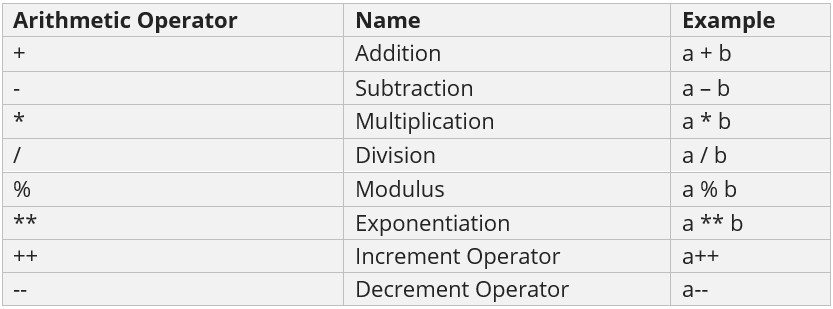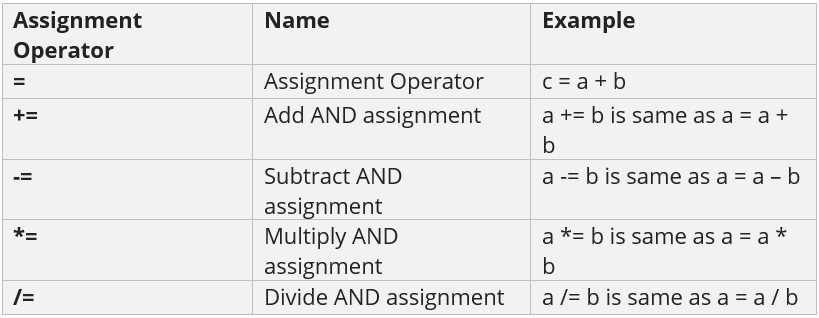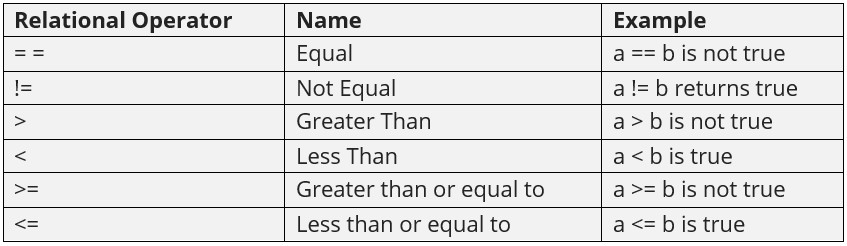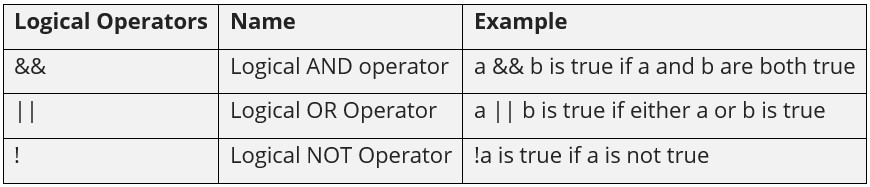## 05 Feb C# Operators

Operators perform operations by taking one or more values, to give another value. These operations are performed on variables and values. Operators in C# are discussed here. For example:

The following are the operators in C#:

• Arithmetic Operators
• Assignment Operators
• Relational Operators
• Logical Operators

Let us understand the operators one by one:

## C# Arithmetic Operators

Arithmetic operators in C# perform arithmetical operations, such as addition, subtraction, division, multiplication, etc.Let us see an example of Arithmetic Operators in C#:

Output

## C# Assignment Operators

Use the Assignment Operator when you need to assign values to a variable. It also includes the Arithmetic Assignment Operators:Let us see an example of Assignment Operators in C#:

Output

## C# Relational Operators

Compare two values with C# relational operators, which are also known as Comparison Operators. Let us say a = 3, b =5;Let us see an example of Relational Operators in C#:

Output

## C# Logical Operators

Logical operators combine conditional statements. Considering Boolean variables, a and b,Let us see an example of Logical Operators in C#:

Output[email protected]

We work to create programming tutorials for all.